# Learning Electronics

Learn to build electronic circuits

# Basic algebra and graphing for electric circuits

### Question 1:

Many different equations used in the analysis of electric circuits may be graphed. Take for instance Ohm's Law for a 1 kW resistor: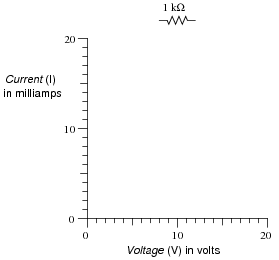Plot this graph, following Ohm's Law. Then, plot another graph representing the voltage/current relationship of a 2 kW resistor.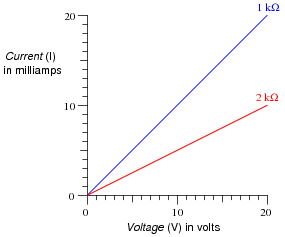Notes:
Ask your students to explain how they plotted the two functions. Did they make a table of values first? Did they draw dots on the paper and then connect those dots with a line? Did anyone plot dots for the endpoints and then draw a straight line in between because they knew this was a linear function?

### Question 2:

Many different equations used in the analysis of electric circuits may be graphed. Take for instance Ohm's Law for a variable resistor connected to a 12 volt source: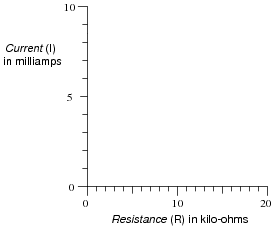Plot this graph, following Ohm's Law.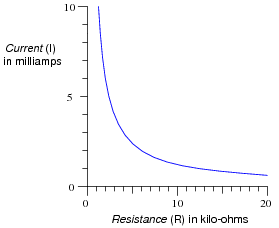Notes:
Ask your students to explain how they plotted the two functions. Did they make a table of values first? Did they draw dots on the paper and then connect those dots with a line? Did anyone plot dots for the endpoints and then draw a straight line in between because they knew this was a linear function?
Many students are surprised that the plot is nonlinear, being that resistors are considered linear devices!

### Question 3:

Observe the following equivalence:

 43 ×42 = (4 ×4 ×4) ×(4 ×4)

Since all operations are the same (multiplication) and reversible, the parentheses are not needed. Therefore, we may write the expression like this:

 4 ×4 ×4 ×4 ×4

Of course, the simplest way to write this is 45, since there are five 4's multiplied together.
Expand each of these expressions so that there are no exponents either:

35 ×32 =
104 ×103 =
82 ×83 =
201 ×202 =

After expanding each of these expressions, re-write each one in simplest form: one number to a power, just like the final form of the example given (45). From these examples, what pattern do you see with exponents of products. In other words, what is the general solution to the following expression?

 am ×an =

 am ×an = am+n

Notes:
I have found that students who cannot fathom the general rule (am ×an = am+n) often understand for the first time when they see concrete examples.

### Question 4:

Observe the following equivalence:

 43 42 = 4 ×4 ×4 4 ×4

It should be readily apparent that we may cancel out two quantities from both top and bottom of the fraction, so in the end we are left with this:

 4 1

Re-writing this using exponents, we get 41.
Expand each of these expressions so that there are no exponents either:
[(35)/(32)] =
[(106)/(104)] =
[(87)/(83)] =
[(205)/(204)] =
After expanding each of these expressions, re-write each one in simplest form: one number to a power, just like the final form of the example given (41). From these examples, what pattern do you see with exponents of products. In other words, what is the general solution to the following expression?

 am an =

 am an = am-n

Notes:
I have found that students who cannot fathom the general rule ([(am)/(an)] = am-n) often understand for the first time when they see concrete examples.

### Question 5:

Observe the following equivalence:

 42 43 = 4 ×4 4 ×4 ×4

It should be readily apparent that we may cancel out two quantities from both top and bottom of the fraction, so in the end we are left with this:

 1 4

Following the rule of [(am)/(an)] = am-n, the reduction of [(42)/(43)] should be 4-1. Many students find this confusing, as the intuitive concept of exponents (how many times a number is to be multiplied by itself) fails here. How in the world do we multiply 4 by itself -1 times?!
Expand each of these expressions so that there are no exponents either:
[(32)/(35)] =
[(104)/(106)] =
[(83)/(87)] =
[(204)/(205)] =
After expanding each of these expressions, re-write each one in simplest form: one number to a power, just like the final form of the example given (4-1), following the rule [(am)/(an)] = am-n. From these examples, what easy-to-understand definition can you think of to describe negative exponents?
Also, expand the following expression so there are no exponents, then re-write the result in exponent form following the rule [(am)/(an)] = am-n:

 53 53

What does this tell you about exponents of zero?
A negative exponent is simply the reciprocal (1/x) of its positive counterpart. A zero exponent is always equal to 1.

Notes:
I have found that students who cannot fathom the meaning of negative or zero exponents often understand immediately when they construct their own definition based on the general rule ([(am)/(an)] = am-n).

### Question 6:

When evaluating (calculating) a mathematical expression, what order should you do the various expressions in? In other words, which comes first: multiplication, division, addition, subtraction, powers, roots, parentheses, etc.; and then what comes after that, and after that?
Do what is inside parentheses first (the furthest ïnside" parentheses if there are multiple layers of parentheses), powers and roots, functions (trig, log, etc.), multiplication/division, and finally addition/subtraction.

Notes:
Order of operations is extremely important, as it becomes critical to recognize proper order of evaluation when ßtripping" an expression down to isolate a particular variable. In essence, the normal order of operations is reversed when ündoing" an expression, so students must recognize what the proper order of operations is.

### Question 7:

Follow proper order of operations to evaluate these expressions:

 13 + 2 3 + 8 =                                        25 + (3 + 2)2 ×2 =

 13 + 2 3 + 8 = 13                             25 + (3 + 2)2 ×2 = 75

Notes:
Nothing special here - just simple arithmetic problems that cannot be solved correctly unless proper order of operations is followed.

### Question 8:

Follow proper order of operations to evaluate these expressions:

 15 - 3 3 + 7 =                                        20 + (1 + 3)2 ×3 =

 15 - 3 3 + 7 = 11                             20 + (1 + 3)2 ×3 = 68

Notes:
Nothing special here - just simple arithmetic problems that cannot be solved correctly unless proper order of operations is followed.

### Question 9:

When evaluating an expression such as this, it is very important to follow proper order of operations. Otherwise, the correct result will be impossible to arrive at:

 3 log25 + 14

To show what the proper order of operations is for this expression, I show it being evaluated step by step heref:

 3 log25 + 14

 3 log32 + 14

 3 ×1.5051 + 14

 4.5154 + 14

 18.5154

Do the same for each of the following expressions:

10 - 25 ×2 + 5
-8 + 103 ×51
124 ×(3 + 11)
21(7 - 4) ×40
log{6 + 352}
{([220/16] - 2.75) ×2}

### Footnotes:

f By the way, this is a highly recommended practice for those struggling with mathematical principles: document each and every step by re-writing the expression. Although it takes more paper and more effort, it will save you from needless error and frustration!
I'll let you determine and document the proper order of operations, but here are the results of each expression:

10 - 25 ×2 + 5 = -35
-8 + 103 ×51 = 50992
124 ×(3 + 11) = 290304
21(7 - 4) ×40 = 370440
log{6 + 352} = 1.5451
{([220/16] - 2.75) ×2} = 4.6904

Notes:
Order of operations is extremely important, as it becomes critical to recognize proper order of evaluation when ßtripping" an expression down to isolate a particular variable. In essence, the normal order of operations is reversed when ündoing" an expression, so students must recognize what the proper order of operations is.

### Question 10:

Perform the following calculations:

 812 810 = 53 54 =                        ( 24 ) ( 2-1 ) =

 812 810 = 64 53 54 = 1 5 = 0.2                  ( 24 ) ( 2-1 ) = 8

Notes:
Nothing special here, just practice with exponents.

### Question 11:

Perform the following calculations:

 106 103 = 32 33 =                        ( 26 ) ( 2-4 ) =

 106 103 = 1000 32 33 = 1 3 � 0.333                  ( 26 ) ( 2-4 ) = 4

Notes:
Nothing special here, just practice with exponents.

### Question 12:

The equation for calculating total resistance in a parallel circuit (for any number of parallel resistances) is sometimes written like this:

 Rtotal = (R1-1 + R2-1 + �Rn-1)-1

Re-write this equation in such a way that it no longer contains any exponents.

Rtotal = 1

 1 R1 + 1 R2 + � 1 Rn

Notes:
This question is an exercise in basic algebra, specifically the meaning of negative exponents.

### Question 13:

A function is a mathematical relationship with an input (usually x) and an output (usually y). Here is an example of a simple function:

 y = 2x + 1

One way to show the pattern of any given function is with a table of numbers. Complete this table for the given values of x:

 x 2x + 1 0 1 2 3 4 5

A more common (and intuitive) way to show the pattern of any given function is with a graph. Complete this graph for the same function y = 2x + 1. Consider each division on the axes to be 1 unit: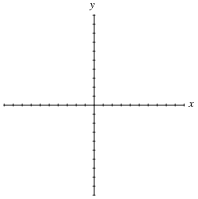x 2x + 1 0 1 1 3 2 5 3 7 4 9 5 11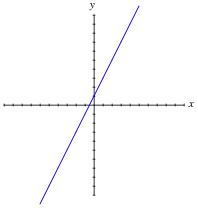Notes:
It is very important for your students to understand graphs, as they are very frequently used to illustrate the behavior of circuits and mathematical functions alike. Discuss with them how the line represents a continuous string of points and not just the integer values calculated in the table.

### Question 14:

A famous illustrative story for understanding exponents goes something like this:
A pauper saves the life of a king. In return, the king offers the pauper anything he desires as a reward. The pauper, being a shrewd man, tells the king he does not want much, only a grain of rice today, then double that (two grains of rice) the next day, then double that (four grains of rice) the next day, and so on. The king asks how long he is to give the pauper rice, and the pauper responds by saying one day for every square on a chess board (64 days). This does not sound like much to the king, who never took a math course, and so he agrees.
In just a short amount of time, though, the king finds himself bankrupted to the pauper because the quantity of rice is so enormously large. Such is the nature of exponential functions: they grow incredibly large with modest gains in x.
Graph the pauper's rice function (y = 2x), with each division on the horizontal axis representing 1 unit and each division on the vertical axis representing 100 units.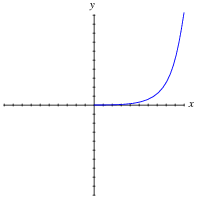Follow-up question: what do you think this graph will look like for negative values of x?

Notes:
From the graph shown, it may appear that the function approaches 0 as x approaches zero. This is not the case, as a simple calculation (y = 20) shows. In order for students to adequately see what is going on near the origin, they will have to re-scale the graph.

### Question 15:

Match each written function (y = ) with the sketched graph it fits best:

 y = 3x + 2                     y = 5 - 2x

 y = x2                     y = 2x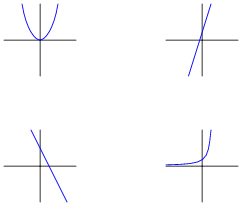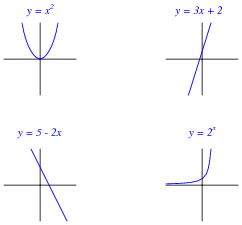Notes:
The primary purpose of this question is to have students figure out how to match each expression to a graph. Of course, one could take the time to plot each function one by one, but there exist much simpler ways to determine the "character" of a function without plotting the whole thing.

### Question 16:

Match each written function (y = ) with the sketched graph it fits best:

 y = 5x - 2                     y = 1 - 3x

 y = x3                     y = 3x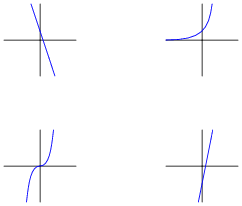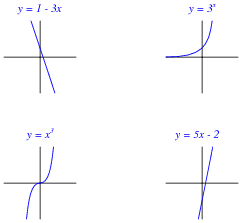Notes:
The primary purpose of this question is to have students figure out how to match each expression to a graph. Of course, one could take the time to plot each function one by one, but there exist much simpler ways to determine the "character" of a function without plotting the whole thing.